Dividing FractionsCustom SearchDIVIDING FRACTIONS The ruler of arithmetic apply to the division of algebraic fractions; as in arithmetic, simply invert the divisor and multiply, as follows: Arithmetic:Algebra: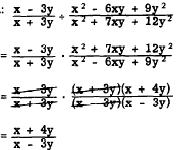Practice problems. In the following problems, divide and reduce to lowest terms:ADDING AND SUBTRACTING FRACTIONS The rules of arithmetic for adding and subtracting fractions are applicable to algebraic fractions. Fractions that are to be combined by addition or subtraction must have the same denominator, The numerators are then combined according to the operation indicated and the result is placed over the denominator. For example, in the expressionthe second denominator will be the same as the first, if its sign is changed, The value of the fraction will remain the same if the sign of the numerator is also changed, Thus, we have the following simplification:When the denominators are not the same, we must reduce all fractions to be added or subtracted to a common denominator and then proceed. Consider, for example,We first must find the least common denominator (LCD). Remember this is the least number that is exactly divisible by each of the denominators. To find such a number, as in arithmetic, we first separate each of the denominators into prime factors. The LCD will contain all of the various prime factors, each one as many times as it occurs in any of the denominators. Factoring, we haveand the LCD is (x + 2)(x - 2)(x - 6). Rewriting the fractions with this denominator and adding numerators, we have the following expression:As another example, consider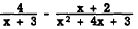Factoring the denominator of the second fraction, we find that the LCD is (x + 3)(x + 1). Rewriting the original fractions with the LCD as denominator, we may now combine the fractions as follows: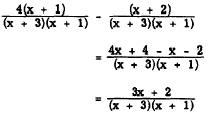Practice problems. Perform the indicated operations in each of the following problems: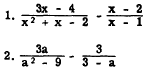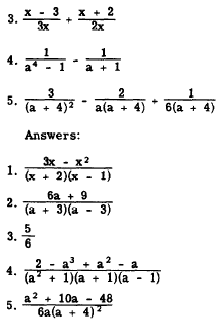Integrated Publishing, Inc. - A (SDVOSB) Service Disabled Veteran Owned Small Business